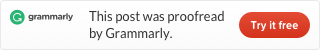Categories

# LeetCode 349. Intersection of Two Arrays

Given two arrays, write a function to compute their intersection.

Example 1:

```Input: nums1 = [1,2,2,1], nums2 = [2,2]
Output: 
```

Example 2:

```Input: nums1 = [4,9,5], nums2 = [9,4,9,8,4]
Output: [9,4]```

Note:

• Each element in the result must be unique.
• The result can be in any order.

First, we use a hashmap to record numbers occur in the first array. Then we count the second array if a number is not in the first array, we don’t put it to the second hashmap either. In the end, all elements in the second hashmap are the results we need.

To see more hashtable related problems, see
Problems and Solutions of Hashmap.This site uses Akismet to reduce spam. Learn how your comment data is processed.